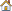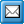﻿ Operators
QPR Knowledge Base 2017.1

OperatorsMetrics User's Guide > Using QPR Metrics > Formulas:

OperatorsOperatorsArithmetic Operators

The following arithmetic operations are available for formula definition:

Description

Addition. Returns the sum of the supplied values. If one or both of the supplied values are text, concatenation of the supplied values is performed (with the possible numeric value first converted into text).

Syntax

X+Y

Parameter: X

Any numeric or text value.

Parameter: Y

Any numeric or text value.

Examples

This operation returns 16:

12+4

This operation returns 111:

"11"+1

This operation returns abcd:

"ab"+"cd"

- (Subtraction)

Description

Subtraction. Returns the difference of the supplied values.

Syntax

X-Y

Parameter: X

Any numeric value.

Parameter: Y

Any numeric value.

Examples

This operation returns 17:

21-4

- (Negation)

Description

Negation. Returns the negation of the supplied value.

Syntax

-X

Parameter: X

Any numeric value.

Examples

This operation returns -67:

-67

* (Multiplication)

Description

Multiplication. Returns the product of the supplied values.

Syntax

X*Y

Parameter: X

Any numeric value.

Parameter: Y

Any numeric value.

Examples

This operation returns 2193:

43*51

/ (Division)

Description

Division. Returns the quotient of the supplied values.

Syntax

X/Y

Parameter: X

Any numeric value.

Parameter: Y

Any non-zero numeric value.

Examples

This operation returns 31:

124/4

DIV

Description

Integer division. Returns the quotient of the supplied values as an integer value.

Syntax

(X) DIV (Y)

Parameter: X

Integer value.

Parameter: Y

Any non-zero integer value.

Examples

This operation returns 1:

(3) DIV (2)

MOD

Description

Modulo. Returns the remainder of the division.

Syntax

(X) MOD (Y)

Parameter: X

Integer value.

Parameter: Y

Any non-zero integer value.

Examples

This operation returns 1:

(3) MOD (2)

^ (Power of)

Description

Power of. Raises the base number X to the power of Y.

Syntax

X ^ Y

Parameter: X

Any numeric value.

Parameter: Y

Any numeric value.

Examples

This operation returns 144:

12 ^ 2

Logical Operators

In logic, a variable or an expression can have only one of two values: TRUE or FALSE.

The following is a list of all the logical operators in alphabetical order and along with an in-depth description of each:

AND

Description

Returns TRUE if the arguments are true; returns FALSE if at least one argument is false.

Syntax

(cond1 ) AND (cond2 )

The two arguments cond1 and cond2 are separated by the logical operator AND.

Examples

This logical operator returns TRUE because both arguments are true:

(1=1) AND (3=3)

NOT

Description

Returns a logical value that is the opposite of the specified value.

Syntax

NOT(logical )

Parameter: logical

An expression that returns a logical value such as TRUE (1) or FALSE (0).

Remarks

If logical  is false, NOT returns True. Conversely, if logical  is true, NOT returns False. This operator is useful together with logical functions like IIF and other logical operators such as AND and OR.

Examples

This function returns False if the "Actual" value (series identifier "ACT") of MEA1 is 1:

NOT(MEA1(ACT) = 1)

OR

Description

Returns TRUE if at least one of the logical arguments is true.

Syntax

(cond1 ) OR (cond2 )

The two arguments cond1 and cond2 are separated by the logical operator OR.

Remarks

This is useful if used together with other logical functions like IIF and NOT and logical operators such as AND.

Examples

This function returns TRUE because one of its arguments is true:

((1 + 1) = 1) OR ((5 + 5) = 10)

XOR

Description

Returns TRUE if either one, but only one, of the logical arguments is true (exclusive OR).

Syntax

(cond1 ) XOR (cond2 )

The two arguments cond1 and cond2 are separated by the logical operator XOR.

Examples

This function returns TRUE because one (and only one) of its arguments is true:

((1 + 1) = 1) XOR ((5 + 5) = 10)

< LESS THAN SIGN

Description

Returns TRUE if the first logical argument is less than the second arguments. For Text series or Text constants, the comparison is performed character by character. When the operator has both a string and a number as arguments, the number is converted to string, after which the string comparison is performed.

Syntax

(cond1 ) < (cond2 )

The two arguments cond1 and cond2 are separated by the logical operator <.

Examples

This function returns TRUE because the first logical argument is less than the second argument:

((5+1)=6) < 10

This function returns FALSE because the first logical argument is more than the second logical argument:

("2") < ("111")

> GREATER THAN SIGN

Description

Returns TRUE if the first logical argument is more than the second arguments. For Text series or Text constants, the comparison is performed character by character. When the operator has both a string and a number as arguments, the number is converted to string, after which the string comparison is performed.

Syntax

(cond1 ) > (cond2 )

The two arguments cond1 and cond2 are separated by the logical operator >.

Examples

This function returns TRUE because the first logical argument is greater than the second argument:

10 > ((5+1)=6)

This function returns TRUE because the first logical argument is greater than the second argument:

("2") > ("111")

<= LESS THAN OR EQUAL TO SIGN

Description

Returns TRUE if the first logical argument is less than or equal to the second arguments. For Text series or Text constants, the comparison is performed character by character. When the operator has both a string and a number as arguments, the number is converted to string, after which the string comparison is performed.

Syntax

(cond1 ) <= (cond2 )

The two arguments cond1 and cond2 are separated by the logical operator <=.

Examples

This function returns TRUE because the first logical argument is less than the second argument:

((5+1)=6) <= 10

This function returns FALSE because the first logical argument is greater than the second argument:

("2") <= ("111")

>= GREATER THAN OR EQUAL TO SIGN

Description

Returns TRUE if the first logical argument is more than or equal to the second arguments. For Text series or Text constants, the comparison is performed character by character. When the operator has both a string and a number as arguments, the number is converted to string, after which the string comparison is performed.

Syntax

(cond1 ) >= (cond2 )

The two arguments cond1 and cond2 are separated by the logical operator >=.

Examples

This function returns TRUE because the first logical argument is more than the second argument:

10 >= ((5+1)=6)

This function returns TRUE because the first logical argument is greater than the second argument:

("2") >= ("111")

<> NOT EQUAL TO SIGN

Description

Returns TRUE if the logical arguments are not equal. For Text series or Text constants, the comparison is performed character by character. When the operator has both a string and a number as arguments, the number is converted to string, after which the string comparison is performed.

Syntax

(cond1 ) <> (cond2 )

The two arguments cond1 and cond2 are separated by the logical operator <>.

Examples

This function returns TRUE because logical arguments are not equal:

10 <> ((5+1)=6)

This function returns TRUE because the logical arguments are not equal:

("2") <> ("111")

= EQUAL TO SIGN

Description

Returns TRUE if the logical arguments are equal. For Text series or Text constants, the comparison is performed character by character. When the operator has both a string and a number as arguments, the number is converted to string, after which the string comparison is performed.

Syntax

(cond1 ) = (cond2 )

The two arguments cond1 and cond2 are separated by the logical operator =.

Examples

This function returns TRUE because arguments are equal:

((5+1)=6) = ((7-1)=6)

This function returns TRUE because the logical arguments are equal:

("abc") = ("abc")

This function returns FALSE because the logical arguments are not equal:

("abc") = ("acb")